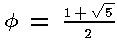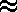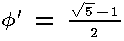# Same Shape but maybe not same Size: Similarity

### Similarity

Scale models are common in many hobbies and professions. Common examples would be cars, ships, planes, trains, rockets, [matreshka] dolls, and figurines, not to mention scale drawings or scale models for architectural plans. Common optics such as binoculars, microscopes, telescopes, and even projection TV's and movie projectors operate under the same principle. In each case there is a preimage and a corresponding image with a certain size change relating them. We can thus define a similarity transformation in terms of the cartesian coordinates of each image point. (For 2-D, let z=0 or omit it.)

 Let Sk be the transformation mapping (x,y,z) onto (kx,ky,kz).

k is variously called the magnitude, scale factor, size change factor, or even ratio of similitude. It can be any value but zero. However, k is usually taken as positive. Negative values result in a 180° rotation of the figure. Unless k=1, a similarity transformation is not an isometry. The isometries (reflection, translation, rotation, and glide reflection), are only one class of transformations. Another important class of transformations are those which preserve shape without necessarily preserving size. A formal definition follows.

 A transformation is a similarity transformation if and only if it is the composition of size changes and reflections.

Remember how isometries preserved Angle measure, Betweenness, Collinearity, and Distance (ABCD Theorem). Angle measure, Betweenness, and Collinearity are still preserved by similarity. Thus only Distance is not preserved. However, distance is scaled by the magnitude k. That is, the distance between any points on the new figure is now k times the original distance. If 0 < k < 1, our resulting image is smaller than the original. This is called a contraction. If k > 1, our resulting image is larger than the original. This is called an expansion, a dilation, or perhaps a dilatation. If k = 1, we have the identity transformation and our resulting image is the same size as the original. A classic example would be enlargements of a picture or letters in different font sizes: zZZZ.

Figures F and G are similar, (notation: F ~ G), if and only if the size change and reflections map one onto the other. Similar Figures have the following properties:

 Segments formed by the points on the pre-image are parallel to the corresponding segment. The distance between two points on the image is k times the distance between the pre-image points. Lines connecting pre-image and the corresponding transformed image points intersect at an origin.

### Similitude in Measurements

If two figures are similar, then corresponding angles are congruent but the sides lengths are proportional. The ratio of similitude for similar figures is useful for relating other measurements, such as perimeter, area, and volume. However, one must keep the dimensionality of the measurement clearly in mind. That is to say, perimeter scales as k, whereas area scales as k2 and volume as k3.

Example: Consider a similarly proportioned 5'6", 67 Kg math teacher and 6'8" basketball player. Find the basketball player's mass. Solution: Their masses correspond not directly with height, but with the cube. Thus to find the corresponding mass of the basketball player we form the proportion: (66"/80")3=67/x. We need to be able to easily solve for x=119 Kg (or 263 pounds). More on that below.

Example: One of the biggest controversies regarding scale models and proportions (see below) involves the infamous Barbie doll. It debutted in 1959, thus has had a long history over several generations, sold over a billion since, and two currently sell each second somewhere. The average American girl will possess eight. Feminists hate her, labelling her a "vacuous blonde." Others blame her for bulimia, anerexia, and teen suicide. In 1998 Mattel released two dozen new Barbies, a third of which had modified body shapes to "appear more contemporary." Perhaps it was in response to complaints about her reputed 38-18-34 dimensions. Thus the modified dolls were thicker waisted, flatter chested, had flatter feet, and were more tight lipped. Consider an old-fashioned Barbie measuring 11.133" tall which you wish to scale up to 5'9". If you have access to a real Barbie, measure her bust, waist, and hips, then compute the corresponding ratios, and compare them with the values of 35-?-31 found by one author.

If G is the pre-image and G' the image, then:
 Perimeter of G' = k1 × perimeter of G. Area of G' = k2 × area of G. Volume of G' = k3 × volume of G.

### Stretch, Shear

Consider now 2×2 matrices under matrix multiplication. We will restrict ourselves to this simple case. Let aij be the arbitrary element in matrix A in row i and column j and bij be the arbitrary element in matrix B in row i and column j. Thus matrix A=
 a11 a12 a21 a22
and matrix B=
 b11 b12 b21 b22
and A × B = C, where c11=a11×b11 + a12×b21,   c12=a11×b12 + a12×b22,   c21=a21×b11 + a22×b21, and   c22=a21×b12 + a22×b22, or matrix C=
 a11×b11 + a12×b21  a11×b12 + a12×b22 a21×b11 + a22×b21  a21×b12 + a22×b22
. This may be easier to see with the dot product of vectors, but we will leave that topic for another day. Specifically, however, please note that unlike matrix addition and subtraction, in matrix multiplication we do not just multiply element by element.

Now consider what happens if the 2×2 identity matrix matrix I2=
 1 0 0 1
is used to either left or right multiply. I2 × A = A × I2 = A.

Continuing, consider what happens when the following matrix is used. With this notation, a size change of 2 can be represented by transforming every point P=(x,y) by multiplying the matrix matrix P=
 x 0 0 y
by the matrix
 2 0 0 2
and a size change of ½ can be similarly transformed using the matrix
 ½ 0 0 ½
. Thus matrices are used extensively for transformations.

### Fixed Points

A fixed point is a point which doesn't change under a given transformation. We can classify isometric transformations by how many fixed points there are. Specifically, if all points are fixed points, then the transformation is the identity transformation. If not all, yet an infinite number of points remain fixed, then we have a reflection over this line of points. If only one point is fixed, then we have a rotation about this point. If no points remain fixed we have a translation. What can you prove if you find three noncollinear fixed points?

### Ratios and Proportions

A ratio is a quotient of two integers that have the same units of measure. Rates are similar to ratios, but the units of measure differ between the two numbers. For example, 60 miles per hour is a rate, whereas 60 mph/30 mph is a ratio. A ratio can be written in several different forms: m:n or m/n (with a solidus) or with the m above the n (with a vinculum). When two ratios (or rates) are equal we can set them equal in a proportion. Proportions are an important way to solve certain types of problems.

When working with proportions, one often encounters what is called cross-multiplication. Before getting into that, let's be clear about the names of the various terms involved in a proportion. Two naming systems are in common use. The first method numbers the terms. Thus if the proportion is a/b=c/d, a is term 1; b is term 2; c is term 3; and d is term 4. The terms numbered with squares (1 and 4) are known as the extremes, whereas the terms numbered with primes (2 and 3) are known as the means.

Cross-multiplication is often taught as a new trick, but really is the same as clearing the denominators, that is, multiplying by both denominators. Thus starting with a/b=c/d, we can multiply both sides by b and by d and obtain ad=cb, where we have cancelled out common factors. We can get there in one step by also multiplying the extremes and setting the product equal to the product of the means. When done in this fashion, we are using the Means-Extremes Property or doing cross multiplication. The point is that this short-cut method is nothing new, and the same concerns about multiplying by something which could be zero apply. When the means are equal (cf problem 12.5#16) we obtain the geometric mean which will be discuss more in the next lesson and was already covered in statistics. Just like we had extended ratios, we can have extended proportions. The law of sines in lesson 7 is a good example.

### The Golden Ratio

Since we introduced the Golden Ratio earlier, we expect you to remember the symbol commonly used (Ø), the value (or1.618...0.618....), the type of number (irrational), the quadratic equation it is associated with (x2 - x - 1 = 0), and how the ratio of consecutive Fibonacci numbers converges alternately from above and below to this number. There is much more that can be said. In fact, whole books (The Golden Ratio by Mario Livio) have been written on the subject. Some consider rectangles with this ratio to have the most aesthetically pleasing shape. The Greeks and perhaps other ancient cultures may have used this ratio in planning their constructions (but perhaps not!). The controversy regarding the Egyptian pyramids is not likely to abate soon.

### Gulliver's Travels

The social satire Gulliver's Travels written by Jonathan Swift and published in 1726 makes extensive use of proportions and also makes a very imaginative children's tale. As you may [or may not] recall, Mr. Gulliver makes four different voyages and encounters four very different cultures. The Lilliputians are 1/12 his size and mired in mistrust and trivial disputes. The Brobdingnagians are 12 times his size, very generous and big-hearted. During the third and fourth voyages, several cultures including the Houyhnhnms and Yahoos, remind Mr. Gulliver of many ruined lives.

The extremes Mr. Gulliver encountered emphasizes the biological limitations of scale. Small creatures can exist with only an exoskeleton, whereas large creatures require the internal structure of a skeleton. Even then, the large legs of an elephant are in sharp contrast with the long neck and narrow legs of a giraffe. Without the bouyancy of water, a blue whale quickly sufficates. Similarly, a mosquito can walk on water, but if hit by a rain drop might never get out of the water alive (awww). (The large mammals which existed after the demise of the dinosaurs may have been aided by an atmosphere with a higher oxygen content than we have today.)

The human body has an average shape, which is getting taller and heavier in recent years, but there is great variation in the size of various parts. Presumably such variation follows the normal (bell-shaped) distribution), but exercise, diet, drugs, and surgery are often used to attain certain shapes deemed more attractive or desireable. As styles change, the task of finding clothes which "fit" becomes challenging as various cracks, crevises, and protuberances are more or less concealed or revealed. In addition to the generational differences, there are cultural differences to which many remain unaware. An interesting project our textbook suggests on page 730 is to use your weight/height to create a chart for similarly proportioned people ranging from 4'6" to 6'6", in two-inch increments. Do standard height/weight charts assume similarity?

music structure (page 690--12.1#19--dominant 7th chord) use graph paper for cube net (page 719) put students in means/extreme proportion. page 725 note kilocalories (not calories)

BACK HOMEWORK ACTIVITY CONTINUE